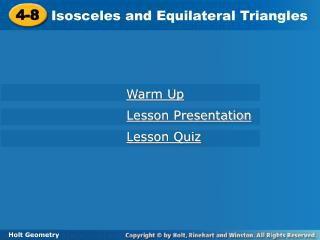DownloadDownload Presentation4-8

# 4-8

Download Presentation## 4-8

- - - - - - - - - - - - - - - - - - - - - - - - - - - E N D - - - - - - - - - - - - - - - - - - - - - - - - - - -
##### Presentation Transcript

1. 4-8 Isosceles and Equilateral Triangles Warm Up Lesson Presentation Lesson Quiz Holt Geometry

2. Do Now • 1. Find each angle measure. • True or False. If false explain. • 2. Every equilateral triangle is isosceles. • 3. Every isosceles triangle is equilateral.

3. Objectives TSW prove theorems about isosceles and equilateral triangles. TSW apply properties of isosceles and equilateral triangles.

4. Vocabulary legs of an isosceles triangle vertex angle base base angles

5. In an Isosceles triangle, the congruent sides are called the legs. The vertex angle is the angle formed by the legs. The side opposite the vertex angle is called the base, and the base angles are the two angles that have the base as a side. 3 is the vertex angle. 1 and 2 are the base angles.

6. Reading Math The Isosceles Triangle Theorem is sometimes stated as “Base angles of an isosceles triangle are congruent.”

7. The length of YX is 20 feet. Explain why the length of YZ is the same. Example 1: Astronomy Application

8. Example 2 If the distance from Earth to a star in September is 4.2  1013 km, what is the distance from Earth to the star in March? Explain. 4.2  1013; since there are 6 months between September and March, the angle measures will be approximately the same between Earth and the star. By the Converse of the Isosceles Triangle Theorem, the triangles created are isosceles, and the distance is the same.

9. Example 5 Find mH.

10. Example 6 Find mN.

11. The following corollary and its converse show the connection between equilateral triangles and equiangular triangles.

12. Example 7: Using Properties of Equilateral Triangles Find the value of x.

13. Example 8: Using Properties of Equilateral Triangles Find the value of y.

14. Example 9 Find the value of JL.

15. Remember! A coordinate proof may be easier if you place one side of the triangle along the x-axis and locate a vertex at the origin or on the y-axis.

16. 1 2 Example 4: Using Coordinate Proof Prove that the segment joining the midpoints of two sides of an isosceles triangle is half the base. Given: In isosceles ∆ABC, X is the mdpt. of AB, and Y is the mdpt. of AC. Prove:XY = AC.

17. By the Midpoint Formula, the coordinates of X are (a, b), and Y are (3a, b). By the Distance Formula, XY = √4a2 = 2a, and AC = 4a. Therefore XY = AC. 1 2 Example 4 Continued Proof: Draw a diagram and place the coordinates as shown.

18. y A(0, 2b) X Y x Z B(–2a, 0) C(2a, 0) Check It Out! Example 4 What if...? The coordinates of isosceles ∆ABC are A(0, 2b), B(-2a, 0), and C(2a, 0). X is the midpoint of AB, and Y is the midpoint of AC. Prove ∆XYZ is isosceles. Proof: Draw a diagram and place the coordinates as shown.

19. By the Midpoint Formula, the coordinates. of X are (–a, b), the coordinates. of Y are (a, b), and the coordinates of Z are (0, 0) . By the Distance Formula, XZ = YZ = √a2+b2 . So XZ YZ and ∆XYZ is isosceles. y A(0, 2b) X Y x Z B(–2a, 0) C(2a, 0) Check It Out! Example 4 Continued

20. Lesson Quiz: Part I Find each angle measure. 1. mR 2. mP Find each value. 3.x 4.y 5.x 28° 124° 6 20 26°

21. Lesson Quiz: Part II 6. The vertex angle of an isosceles triangle measures (a + 15)°, and one of the base angles measures 7a°. Find a and each angle measure. a = 11; 26°; 77°; 77°